University Physics Volume 3

# Chapter 2

### Conceptual Questions

1.

Virtual image cannot be projected on a screen. You cannot distinguish a real image from a virtual image simply by judging from the image perceived with your eye.

3.

Yes, you can photograph a virtual image. For example, if you photograph your reflection from a plane mirror, you get a photograph of a virtual image. The camera focuses the light that enters its lens to form an image; whether the source of the light is a real object or a reflection from mirror (i.e., a virtual image) does not matter.

5.

No, you can see the real image the same way you can see the virtual image. The retina of your eye effectively serves as a screen.

7.

The mirror should be half your size and its top edge should be at the level of your eyes. The size does not depend on your distance from the mirror.

9.

when the object is at infinity; see the mirror equation

11.

Yes, negative magnification simply means that the image is upside down; this does not prevent the image from being larger than the object. For instance, for a concave mirror, if distance to the object is larger than one focal distance but smaller than two focal distances the image will be inverted and magnified.

13.

15.

The focal length of the lens is fixed, so the image distance changes as a function of object distance.

17.

Yes, the focal length will change. The lens maker’s equation shows that the focal length depends on the index of refraction of the medium surrounding the lens. Because the index of refraction of water differs from that of air, the focal length of the lens will change when submerged in water.

19.

A relaxed, normal-vision eye will focus parallel rays of light onto the retina.

21.

A person with an internal lens will need glasses to read because their muscles cannot distort the lens as they do with biological lenses, so they cannot focus on near objects. To correct nearsightedness, the power of the intraocular lens must be less than that of the removed lens.

23.

Microscopes create images of macroscopic size, so geometric optics applies.

25.

The eyepiece would be moved slightly farther from the objective so that the image formed by the objective falls just beyond the focal length of the eyepiece.

### Problems

27.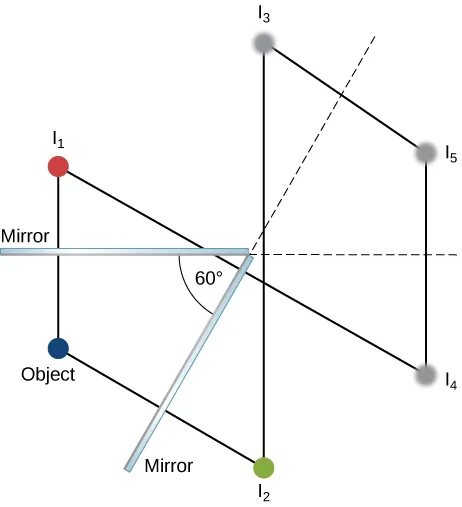29.

It is in the focal point of the big mirror and at the center of curvature of the small mirror.

31.

$f = R 2 ⇒ R = + 1.60 m f = R 2 ⇒ R = + 1.60 m$

33.

$d o = 27.3 cm d o = 27.3 cm$

35.

Step 1: Image formation by a mirror is involved.
Step 2: Draw the problem set up when possible.
Step 3: Find f.
Step 4: Given: $m=1.50,do=0.120mm=1.50,do=0.120m$.
Step 5: No ray tracing is needed.
Step 6: Using the mirror equation, Equation 2.3, $m=dido,di=–mdo=–(1.50)(0.120m)=–0.180mm=dido,di=–mdo=–(1.50)(0.120m)=–0.180m$. Then,
$f=(1do+1di)−1 =(1–0.180m+10.120M)–1=0.360mf=(1do+1di)−1=(1–0.180m+10.120M)–1=0.360m$.
Step 7: The image is virtual because the image distance is negative. The focal length is positive, so the mirror is concave.

37.

a. for a convex mirror $di<0⇒m>0.m=+0.111di<0⇒m>0.m=+0.111$; b. $di=−0.334cmdi=−0.334cm$ (behind the cornea);
c.

39.

$m = h i h o = − d i d o = − − d o d o = d o d o = 1 ⇒ h i = h o m = h i h o = − d i d o = − − d o d o = d o d o = 1 ⇒ h i = h o$

41.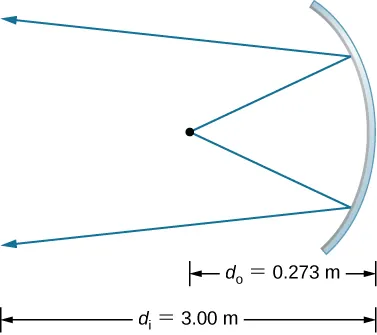$m=−11.0A′=0.110m2I=6.82kW/m2m=−11.0A′=0.110m2I=6.82kW/m2$

43.

$x 2 m = − x 2 m − 1 , ( m = 1 , 2 , 3 , ... ) , x 2 m + 1 = b − x 2 m , ( m = 0 , 1 , 2 , ... ) , with x 0 = a . x 2 m = − x 2 m − 1 , ( m = 1 , 2 , 3 , ... ) , x 2 m + 1 = b − x 2 m , ( m = 0 , 1 , 2 , ... ) , with x 0 = a .$

45.

$d i = −55 cm ; m = + 1.8 d i = −55 cm ; m = + 1.8$

47.

$d i = −41 cm, m = 1.4 d i = −41 cm, m = 1.4$

49.

proof

51.

a. $1di+1do=1f⇒di=3.43m1di+1do=1f⇒di=3.43m$;
b. $m=−33.33m=−33.33$, so that $(2.40×10−2m)(33.33)=80.0cm, and(3.60×10−2m)(33.33)=1.20m⇒0.800m×1.20m or80.0cm×120cm(2.40×10−2m)(33.33)=80.0cm, and(3.60×10−2m)(33.33)=1.20m⇒0.800m×1.20m or80.0cm×120cm$

53.

a. $1do+1di=1fdi=5.08cm1do+1di=1fdi=5.08cm$;
b. $m=−1.695×10−2m=−1.695×10−2$, so the maximum height is $0.036m1.695×10−2=2.12m⇒100%0.036m1.695×10−2=2.12m⇒100%$;
c. This seems quite reasonable, since at 3.00 m it is possible to get a full length picture of a person.

55.

a. $1do+1di=1f⇒do=2.55m1do+1di=1f⇒do=2.55m$;
b. $hiho=−dido⇒ho=1.00mhiho=−dido⇒ho=1.00m$

57.

a. Using $1do+1di=1f1do+1di=1f$, $di=−56.67cmdi=−56.67cm$. Then we can determine the magnification, $m=6.67m=6.67$. b. $di=−190cmdi=−190cm$ and $m=+20.0m=+20.0$; c. The magnification m increases rapidly as you increase the object distance toward the focal length.

59.

$1 d o + 1 d i = 1 f d i = 1 ( 1 / f ) − ( 1 / d o ) d i d o = 6.667 × 10 −13 = h i h o h i = −0.933 mm 1 d o + 1 d i = 1 f d i = 1 ( 1 / f ) − ( 1 / d o ) d i d o = 6.667 × 10 −13 = h i h o h i = −0.933 mm$

61.

$di=−6.7cmdi=−6.7cm$
$hi=4.0cmhi=4.0cm$

63.

83 cm to the right of the converging lens, $m=−2.3,hi=6.9cmm=−2.3,hi=6.9cm$

65.

$P = 52.0 D P = 52.0 D$

67.

$h i h o = − d i d o ⇒ h i = − h o ( d i d o ) = − ( 3.50 mm ) ( 2.00 cm 30.0 cm ) = −0.233 mm h i h o = − d i d o ⇒ h i = − h o ( d i d o ) = − ( 3.50 mm ) ( 2.00 cm 30.0 cm ) = −0.233 mm$

69.

a. $P=+62.5DP=+62.5D$;
b. $hiho=−dido⇒hi=−0.250mmhiho=−dido⇒hi=−0.250mm$;
c. $hi=−0.0800mmhi=−0.0800mm$

71.

$P = 1 d o + 1 d i ⇒ d o = 28.6 cm P = 1 d o + 1 d i ⇒ d o = 28.6 cm$

73.

Originally, the close vision was 51.0 D. Therefore, $P=1do+1di⇒do=1.00mP=1do+1di⇒do=1.00m$

75.

originally, $P=70.0DP=70.0D$; because the power for normal distant vision is 50.0 D, the power should be decreased by 20.0 D

77.

$P = 1 d o + 1 d i ⇒ d o = 0.333 m P = 1 d o + 1 d i ⇒ d o = 0.333 m$

79.

a. $P=52.0DP=52.0D$;
b. $P′=56.16D1do+1di=P⇒do=16.2cmP′=56.16D1do+1di=P⇒do=16.2cm$

81.

We need $di=−18.5cmdi=−18.5cm$ when $do=∞do=∞$, so
$P=−5.41DP=−5.41D$

83.

Let $xx$ = far point
$⇒P=1−(x−0.0175m)+1∞⇒−xP+(0.0175m)P=1⇒x=26.8cm⇒P=1−(x−0.0175m)+1∞⇒−xP+(0.0175m)P=1⇒x=26.8cm$

85.

$M = 6 × M = 6 ×$

87.

$M=(25cmL)(1+L−ℓf) 1do+1–13.1=12.1 do=1.81cm M=(25cmL)(1+L−ℓf) 1do+1–13.1=12.1 do=1.81cm$

89.

$M = 2.5 × M = 2.5 ×$

91.

$M = −2.1 × M = −2.1 ×$

93.

$M = 25 cm f M max = 5 M = 25 cm f M max = 5$

95.

$M max young = 1 + 18 cm f ⇒ f = 18 cm M max young − 1 M max old = 9.8 × M max young = 1 + 18 cm f ⇒ f = 18 cm M max young − 1 M max old = 9.8 ×$

97.

a. $1do+1di=1f⇒di=4.65cm⇒m=−30.01do+1di=1f⇒di=4.65cm⇒m=−30.0$;
b. $Mnet=−240Mnet=−240$

99.

a. $1doobj+1diobj=1fobj⇒diobj=18.3cm1doobj+1diobj=1fobj⇒diobj=18.3cm$ behind the objective lens;
b. $mobj=−60.0mobj=−60.0$;
c. $doeye=1.70cmdieye=−11.3cmdoeye=1.70cmdieye=−11.3cm$
in front of the eyepiece; d. $Meye=13.5Meye=13.5$;
e. $Mnet=−810Mnet=−810$

101.

$M = −40.0 M = −40.0$

103.

$f obj = R 2 , M = −1.67 f obj = R 2 , M = −1.67$

105.

$M = − f obj f eye , f eye = + 10.0 cm M = − f obj f eye , f eye = + 10.0 cm$

107.

109.

12 cm to the left of the mirror, $m=3/5m=3/5$

111.

27 cm in front of the mirror, $m=0.6,hi=1.76cmm=0.6,hi=1.76cm$, orientation upright

113.

The following figure shows three successive images beginning with the image $Q1Q1$ in mirror $M1M1$. $Q1Q1$ is the image in mirror $M1M1$, whose image in mirror $M2M2$ is $Q12Q12$ whose image in mirror $M1M1$ is the real image $Q121Q121$.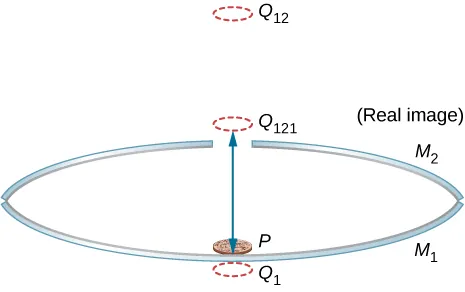115.

5.4 cm from the axis

117.

Let the vertex of the concave mirror be the origin of the coordinate system. Image 1 is at −10/3 cm (−3.3 cm), image 2 is at −40/11 cm (−3.6 cm). These serve as objects for subsequent images, which are at −310/83 cm (−3.7 cm), −9340/2501 cm (−3.7 cm), −140,720/37,681 cm (−3.7 cm). All remaining images are at approximately −3.7 cm.

119.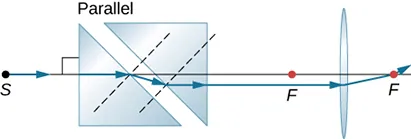121.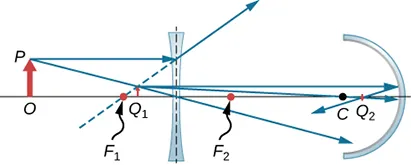123.

−5 D

125.

11

127.

a.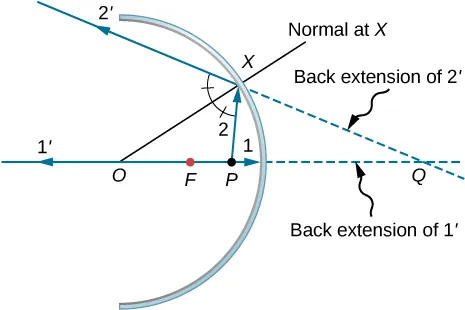b.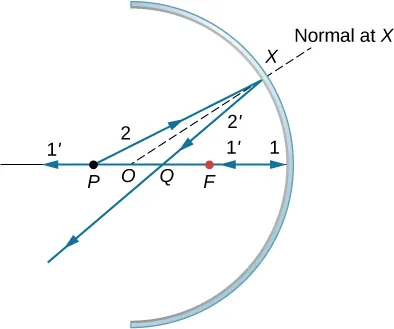c.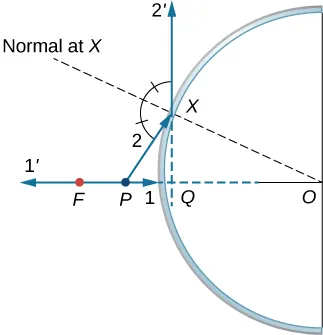d. similar to the previous picture but with point P outside the focal length; e. Repeat (a)–(d) for a point object off the axis. For a point object placed off axis in front of a concave mirror corresponding to parts (a) and (b), the case for convex mirror left as exercises.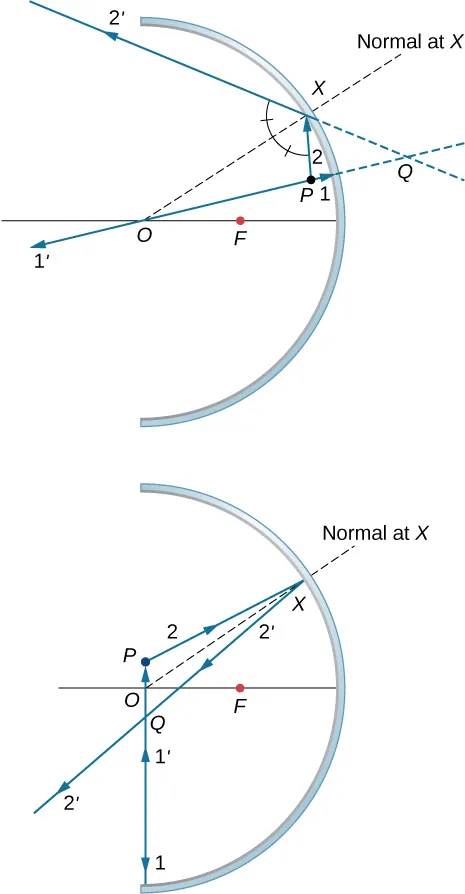129.

$di=−10/3cm,hi=2cmdi=−10/3cm,hi=2cm$, upright

131.

proof

133.Triangles BAO and $B1A1OB1A1O$ are similar triangles. Thus, $A1B1AB=didoA1B1AB=dido$.Triangles NOF and $B1A1FB1A1F$ are similar triangles. Thus, $NOf=A1B1di−fNOf=A1B1di−f$. Noting that $NO=ABNO=AB$ gives $ABf=A1B1di−fABf=A1B1di−f$ or $ABA1B1=fdi−fABA1B1=fdi−f$. Inverting this gives $A1B1AB=di−ff.A1B1AB=di−ff.$ Equating the two expressions for the ratio $A1B1ABA1B1AB$ gives $dido=di−ffdido=di−ff$. Dividing through by $didi$ gives $1do=1f−1di1do=1f−1di$ or $1do+1di=1f1do+1di=1f$.

135.

70 cm

137.

The plane mirror has an infinite focal point, so that $di=−dodi=−do$. The total apparent distance of the man in the mirror will be his actual distance, plus the apparent image distance, or $do+(−di)=2dodo+(−di)=2do$. If this distance must be less than 20 cm, he should stand at $do=10cmdo=10cm$.

139.

Here we want $do=25cm−2.20cm=0.228mdo=25cm−2.20cm=0.228m$. If $x=x=$ near point, $di=−(x−0.0220m)di=−(x−0.0220m)$. Thus, $P=1do+1di=10.228m–1x−0.0220mP=1do+1di=10.228m–1x−0.0220m$. Using $P=0.75DP=0.75D$ gives $x=0.297mx=0.297m$, so the near point is 29.7 cm.

141.

Assuming a lens at 2.00 cm from the boy’s eye, the image distance must be $di=−(500cm−2.00cm)=−498cm.di=−(500cm−2.00cm)=−498cm.$ For an infinite-distance object, the required power is $P=1di=−0.200DP=1di=−0.200D$. Therefore, the $−4.00D−4.00D$ lens will correct the nearsightedness.

143.

$87 μm 87 μm$

145.

Use, $Mnet=−diobj(feye+25cm)fobjfeyeMnet=−diobj(feye+25cm)fobjfeye$. The image distance for the objective is $diobj=−Mnetfobjfeyefeye+25cmdiobj=−Mnetfobjfeyefeye+25cm$. Using $fobj=3.0cm,feye=10cm,andM=−10fobj=3.0cm,feye=10cm,andM=−10$ gives $diobj=8.6cmdiobj=8.6cm$. We want this image to be at the focal point of the eyepiece so that the eyepiece forms an image at infinity for comfortable viewing. Thus, the distance d between the lenses should be $d=feye+diobj=10cm+8.6cm=19cmd=feye+diobj=10cm+8.6cm=19cm$.

147.

a. focal length of the corrective lens $fc=−80cmfc=−80cm$; b. −1.25 D

149.

$2 × 10 16 km 2 × 10 16 km$

151.

$10 5 m 10 5 m$

Order a print copy

As an Amazon Associate we earn from qualifying purchases.